﻿ The MSC Adams/View and Simulation of the Crank Rocker Mechanism

The MSC Adams/View and Simulation of the Crank Rocker Mechanism

Patrik Šarga, Darina Hroncová, Róbert Rákay

American Journal of Mechanical Engineering

The MSC Adams/View and Simulation of the Crank Rocker Mechanism

Patrik Šarga1,, Darina Hroncová1, Róbert Rákay2

1Department of Mechatronics, Technical University of Košice, Faculty of Mechanical Engineering, Letná 9, 042 00 Košice, Slovak republic

2Department of Automation, Control and Human Machine Interactions, Technical University of Košice, Faculty of Mechanical Engineering, Letná 9, 042 00 Košice, Slovak republic

Abstract

In the paper we show the possibilities of physical modeling in MSC Adams of the crank mechanism. The aim of this article is to develop a functional model of crank rocker mechanism in ADAMS/View software and his following complete kinematics analysis. We analyze the movement of the members of the mechanism. Kinematics analysis was performed analytically and graphically. Finally, the work presents the results with graphical representation of parameters such as angular velocity and angular acceleration of the members of the mechanism.

• Patrik Šarga, Darina Hroncová, Róbert Rákay. The MSC Adams/View and Simulation of the Crank Rocker Mechanism. American Journal of Mechanical Engineering. Vol. 3, No. 6, 2015, pp 161-164. http://pubs.sciepub.com/ajme/3/6/1
• Šarga, Patrik, Darina Hroncová, and Róbert Rákay. "The MSC Adams/View and Simulation of the Crank Rocker Mechanism." American Journal of Mechanical Engineering 3.6 (2015): 161-164.
• Šarga, P. , Hroncová, D. , & Rákay, R. (2015). The MSC Adams/View and Simulation of the Crank Rocker Mechanism. American Journal of Mechanical Engineering, 3(6), 161-164.
• Šarga, Patrik, Darina Hroncová, and Róbert Rákay. "The MSC Adams/View and Simulation of the Crank Rocker Mechanism." American Journal of Mechanical Engineering 3, no. 6 (2015): 161-164.

 Import into BibTeX Import into EndNote Import into RefMan Import into RefWorks

1234
Prev Next

1. Introduction

Computer modeling is an effective tool to accelerate and improve the design of new mechanical systems. The MSC Adams/View  is appropriate software tools for creating computer models. In addressing the motion of machine parts, machines and equipment it is necessary first to create a kinematics model. Conventional numerical solution uses vector calculus as the basic mathematical apparatus because the main kinematics variables are vector quantities. Kinematics model of a device schematically captures all its properties which are essential in kinematical analysis i.e. individual members with dimensions, kinematics pairs, and so on. Classical numerical solution of movement kinematics is often lengthy and difficult especially for complex kinematics models with different movements. To simplify and expedite the analysis a graphical solution may be used which is nowadays replaced with a solution using computer techniques using various software products. These software products ease the investigator’s effort. Investigator enters the model configuration and the input data and the program calculates the required outputs. These data can be presented in a tabular form or the program draws graphical results.

2. Description of the Model

MSC Adams is one of the most widely used multi-function computing software . The aim of this article is to create a functional model crank rocker mechanism in ADAMS/View and to make its complete kinematics analysis . The aim is to investigate the movement of individual members of the mechanism and its points.

We analyze the movement of point A and point B on the member 3 (Figure 1). The member 3 is performing planar motion. The point A of the member 2 moves in a circle with radius 12 and point B of the member 4 also moves in circle with radius 14. We determine angular velocity and angular acceleration of member 2, angular velocity and angular acceleration of member 4.

Kinematical analysis of the mechanism means to solve kinematical variables of movement of the driven members with respect to the kinematics variables of movement of the driving members .Download asVeiw figureFigures index
Figure 1. Crank Rocker Mechanism

Respective lengths of the mechanism are: l2=120 mm, l3= 250 mm, l4=260 mm, O21O41=300 mm. Driving link O21A has a counterclockwise angular velocity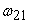=1 (rad/s). Our task is to determine angular displacement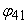, angular velocity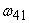and angular acceleration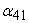of the link O41B graphically for the crank position indicated and then to create a model of a mechanism in MSC Adams/View environment.

The crank O21A rotates around point O21, motion of the connecting member AB is a general planar motion and member O41B rotates around point O41. When we determine lengths of the members we indicate velocities of the points A and B. The angular velocity of the member 2 is.

3. Kinematics Analysis of the Model

The velocity of points A and B can be obtained by using the rule of the viewing angles . Tangent of the angle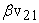under which we see the endpoints of velocity from the permanent center of rotation is proportional to the angular velocity of the rotating member. If we denote the angular velocityof the member 2 with respect to 4, then for the velocity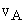and acceleration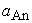at point A shall apply :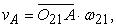(1)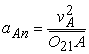(2)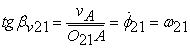(3)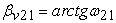(4)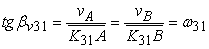(5)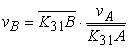(6)

Angular velocityof the member 4 we determine: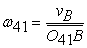(7)

The member 3 is moving in the plane in form 3:1=3:2+2:1 and also 3:1=3:4+4:1. For the velocity of the point A: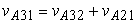(8)

For the velocity of the point B: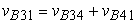(9)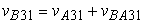(10)

Acceleration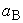of the point B :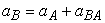(11)

where: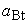- known direction,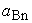- can be obtained by Euclidean construction,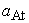- is zero because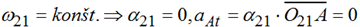(12)– is normal component of the acceleration of the point A:(13)

Graphical solution of the above vector equation is in the Figure 2. Angular velocityand angular accelerationis then calculated according to:(14)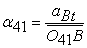(15)

In Figure 2 there is the model in graphic form with velocities of points A and B.Download asVeiw figureFigures index
Figure 2. Crank Rocker Mechanism - velocity of the point A and velocity of the point B

4. Simulation of the Crank Rocker Mechanism Using MSC Adams/View

The given crank rocker mechanism was modeled in MSC ADAMS/View and the initial parameters were provided . In the initial window of the program we set data folder, name of the project, units and the working grid. We created the individual bodies of the mechanism. We selected the rigid body link from the Toolbox. We defined the geometry, length, width and depth. The lengths of the four links crank rocker mechanism are: l2=120 mm, l3=250 mm, l4=260 mm, O21O41=300 mm. Driving link O21A (Figure 1) has a counterclockwise angular velocity=1 rad/s.

We created the member 2 and the member 4 shown in the Figure 3 and Figure 4.Download asVeiw figureFigures index
Figure 3. Creation of the member 2 of the crank rocker

We select link tool in Main Toolbox. Move the cursor to (0, 0, 0) position and member 2 is created a named automatically. Then move the cursor to (300, 0, 0) position and created the member 4.Download asVeiw figureFigures index
Figure 4. Creation of the member 4 of the crank rocker mechanism

The member 4 should be rotated about point from horizontal position to counterclockwise rotated 114° (Figure 5).Download asVeiw figureFigures index
Figure 5. Rotation of the member 4

Then connected the member 2 and 4 with member 3 (Figure 6).Download asVeiw figureFigures index
Figure 6. Connecting member 2 and member 4 with member 3

Next we created joints between the members and ground (Figure 7).Download asVeiw figureFigures index
Figure 7. Connecting members with joints

Select rotational joint motion tool with speed 1 rad/s  (Figure 8).Download asVeiw figureFigures index
Figure 8. Create the rotation joint motion in point O21

Final model of the mechanism is shown in Figure 9.Download asVeiw figureFigures index
Figure 9. Model of the crank rocker mechanism in Adams View

We create the measure windows for angle, angular velocityand angular accelerationof the member 4. Then we start simulation and we determine angle, angular velocityand angular accelerationof the member 4 (Figure 10).Download asVeiw figureFigures index
Figure 10. Illustration of the windows measures for angle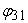, angular velocity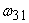and angular acceleration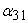of the member 3Download asVeiw figureFigures index
Figure 11. Window of the Postprocessor with animation window and measure windows

In the window of the Postprocessor we selected window with animation of the mechanism and windows with results data in graphics form for angle, angular velocityand angular accelerationof the member 4 (Figure 11).

5. Conclusion

The MSC ADAMS/View software products is program suitable for kinematics analysis that allows to modeled kinematics chains and solve their motion.

We investigated a functional model of a crank rocker mechanism. The whole modeling simulation was carried out by simulation program MSC ADAMS/View. Subsequent simulation of several configurations of the crank rocker mechanism and the comparison of the outputs showed the impact of the change of the respective parameter.

In the work is shown a procedure for solving kinematics problem of the mechanism using analytical and graphical solution. Results are obtained in form of time diagram of the desired variables. The results obtained by the simulation of the crank rocker mechanism were processed by the postprocessor program of MSC Adams/View. 

Program MSC Adams/View makes it easy to analyze complex mechanical systems with multiple degrees of freedom. In the paper one module of the number of modules namely MSC Adams/View was used as a tool which allows a better simulation and visualization of the model and easier evaluation of the results obtained. With the module MSC Adams/View they represent a tool that is able to addresses various types of mechanisms with many degrees of freedom. Mastering this methodology provides a suitable tool for solving problems of teaching and practice.

Acknowledgement

This contribution is a result of the project Slovak Grant Agency – project KEGA 048TUKE-4/2014 “Increasing of knowledge base of students in area of application of embedded systems in mechatronic systems“ and grant projects VEGA No. 1/1205/12 and VEGA No. 1/0393/14.

References

  Hajžman, M.: Pomocný text k proniknutí do základů práce se systémem ADAMS: Information on: http://www.kme.zcu.cz/mhajzman/download/adams_zaklad.pdf.In article  Gmiterko, A., 2004. Mechatronics, Emilenia, Košice, Slovakia.In article  Gmiterko, A., Šarga, P., Hroncová, D., 2012. Mechatronika I, Technická univerzita Košice, Košice, Slovakia.In article  Hroncová, D., 2012. Studies of compatible software tools for abstract and concrete design of mechatronic systems and linking them for the purpose of logical and physical modeling, Dissertation thesis, Košice, Slovakia.In article  Karban, P., 2006. Výpočty a simulace v programech Matlab a Simulink. Computer Press, Brno, Česko.In article  Virgala, I., Frankovský, P., Kenderová, M.: Friction Effect Analysis of a DC Motor. In: American Journal of Mechanical Engineering. 2013. Vol. 1, no. 1 (2013), p. 1-5.In article View Article  Zubkova Y., Turygin Y., Experimental Investigation of Dynamic Error for Manipulator Output Link During Its Motion Path Tryout, Acta Mechanica Slovaca. Volume 19, Issue 3, Pages 34-37.In article